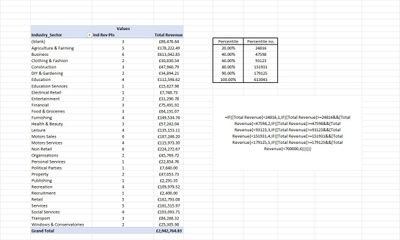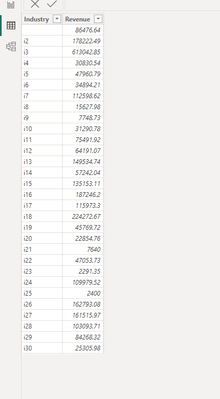cancel
Showing results for
Did you mean:Frequent Visitor

## Dynamic percentile of pivot revenue measureHello, I have a dataset that I isolate by industry types and customers. Operating in excel office 365

I'm looking at recreating industy revenue points dynamically based on what is in the pivot table so that if I filter by month or quarter etc the percentiles change within the measure. Currently I have taken all values of revenue manually and plugged them into a measure manually as shown in the image. Scoring 1 if under 20% percentile, 2 if between 24816 and 47598 etc up to 6 point. I want to then translate that across to customers instead of industries

is this possible?

1 ACCEPTED SOLUTIONCommunity Support

Hi , @king4646

According to your description, you want to "Dynamic percentile of pivot revenue measure".

Here are the steps you can refer to :
(1)This is my test data:(2)We can create a measrue like this:

``````Measure = var _20= PERCENTILEX.INC(ADDCOLUMNS( ALLSELECTED('Table'[Industry]) ,"Sum of Revenue" , CALCULATE(SUM('Table'[Revenue]))) , [Sum of Revenue] , 0.2)
var _40 = PERCENTILEX.INC(ADDCOLUMNS( ALLSELECTED('Table'[Industry]) ,"Sum of Revenue" , CALCULATE(SUM('Table'[Revenue]))) , [Sum of Revenue] , 0.4)
var _60 = PERCENTILEX.INC(ADDCOLUMNS( ALLSELECTED('Table'[Industry]) ,"Sum of Revenue" , CALCULATE(SUM('Table'[Revenue]))) , [Sum of Revenue] , 0.6)
var _80 = PERCENTILEX.INC(ADDCOLUMNS( ALLSELECTED('Table'[Industry]) ,"Sum of Revenue" , CALCULATE(SUM('Table'[Revenue]))) , [Sum of Revenue] , 0.8)
var _90 = PERCENTILEX.INC(ADDCOLUMNS( ALLSELECTED('Table'[Industry]) ,"Sum of Revenue" , CALCULATE(SUM('Table'[Revenue]))) , [Sum of Revenue] , 0.9)
var _100 = PERCENTILEX.INC(ADDCOLUMNS( ALLSELECTED('Table'[Industry]) ,"Sum of Revenue" , CALCULATE(SUM('Table'[Revenue]))) , [Sum of Revenue] , 1)
var _revenue = SUM('Table'[Revenue])
return
IF( _revenue < _20 ,1, IF(_revenue >= _20 &&_revenue<_40 ,2 ,IF( _revenue >=_40 && _revenue<_60 ,3, IF(_revenue>=_60 && _revenue < _80 , 4 , IF(_revenue>=_80 && _revenue < _90 ,5 , 6)))))``````

(3)Then we can put it on the visual , the result is as follows:If this method does not meet your needs, you can provide us with your special sample data and the desired output sample data in the form of tables, so that we can better help you solve the problem. (You can also upload you sample .pbix [without sensitive data] to the OneDrive and share with the OneDrive link to me ! )

Thank you for your time and sharing, and thank you for your support and understanding of PowerBI!

Best Regards,

Aniya Zhang

If this post helps, then please consider Accept it as the solution to help the other members find it more quickly

4 REPLIES 4Community Support

Hi , @king4646

According to your description, you want to "Dynamic percentile of pivot revenue measure".

Here are the steps you can refer to :
(1)This is my test data:(2)We can create a measrue like this:

``````Measure = var _20= PERCENTILEX.INC(ADDCOLUMNS( ALLSELECTED('Table'[Industry]) ,"Sum of Revenue" , CALCULATE(SUM('Table'[Revenue]))) , [Sum of Revenue] , 0.2)
var _40 = PERCENTILEX.INC(ADDCOLUMNS( ALLSELECTED('Table'[Industry]) ,"Sum of Revenue" , CALCULATE(SUM('Table'[Revenue]))) , [Sum of Revenue] , 0.4)
var _60 = PERCENTILEX.INC(ADDCOLUMNS( ALLSELECTED('Table'[Industry]) ,"Sum of Revenue" , CALCULATE(SUM('Table'[Revenue]))) , [Sum of Revenue] , 0.6)
var _80 = PERCENTILEX.INC(ADDCOLUMNS( ALLSELECTED('Table'[Industry]) ,"Sum of Revenue" , CALCULATE(SUM('Table'[Revenue]))) , [Sum of Revenue] , 0.8)
var _90 = PERCENTILEX.INC(ADDCOLUMNS( ALLSELECTED('Table'[Industry]) ,"Sum of Revenue" , CALCULATE(SUM('Table'[Revenue]))) , [Sum of Revenue] , 0.9)
var _100 = PERCENTILEX.INC(ADDCOLUMNS( ALLSELECTED('Table'[Industry]) ,"Sum of Revenue" , CALCULATE(SUM('Table'[Revenue]))) , [Sum of Revenue] , 1)
var _revenue = SUM('Table'[Revenue])
return
IF( _revenue < _20 ,1, IF(_revenue >= _20 &&_revenue<_40 ,2 ,IF( _revenue >=_40 && _revenue<_60 ,3, IF(_revenue>=_60 && _revenue < _80 , 4 , IF(_revenue>=_80 && _revenue < _90 ,5 , 6)))))``````

(3)Then we can put it on the visual , the result is as follows:If this method does not meet your needs, you can provide us with your special sample data and the desired output sample data in the form of tables, so that we can better help you solve the problem. (You can also upload you sample .pbix [without sensitive data] to the OneDrive and share with the OneDrive link to me ! )

Thank you for your time and sharing, and thank you for your support and understanding of PowerBI!

Best Regards,

Aniya Zhang

If this post helps, then please consider Accept it as the solution to help the other members find it more quicklyFrequent Visitor

Sorry for the delay I was on holiday. Thank you very much! It worked perfectly in that situation however when I try to adapt to a customer level it hasn't worked. Could you please assist further?

my sample excel file is on the link belowCommunity Support

Hi , @king4646

Thanks for your response!According to your description, when you  adapt to a customer level it hasn't worked.

And i try to access your url , it return 401 and i have no access to it .

For this , you can try to replace all the "'Table'[Industry]" to 'Table'[customer] if it can help?

Thank you for your time and sharing, and thank you for your support and understanding of PowerBI!

Best Regards,

Aniya Zhang

If this post helps, then please consider Accept it as the solution to help the other members find it more quicklyFrequent Visitor

can any pros help @Greg_Deckler @tamerj1 @FreemanZ @johnt75